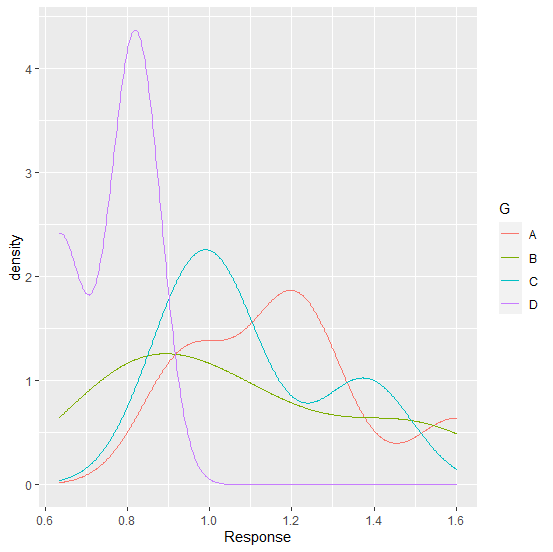# How to create smooth density curves without filling densities in R?

The density curves can be created by using stat_density function of ggplot2 package but it fills the curve with density hence it becomes difficult to recognize the curves. We can remove these densities by using geom="line" inside the stat_density function so that only the density curves will be plotted.

## Example

Consider the below data frame:

Live Demo

> G<-sample(LETTERS[1:4],20,replace=TRUE)
> Response<-rnorm(20,1,0.34)
> df<-data.frame(G,Response)
> df

## Output

G Response
1 C 1.0229016
2 C 1.0058160
3 B 0.8831558
4 B 0.7729167
5 C 0.9130468
6 D 0.8431893
7 B 1.5003581
8 A 0.9687335
9 B 1.1139661
10 A 0.9211660
11 A 1.1790619
12 D 0.6349671
13 A 1.2616918
14 A 1.6021078
15 C 0.9332981
16 C 1.0696149
17 D 0.7971567
18 C 1.4006042
19 C 1.3568032
20 A 1.1907336

> library(ggplot2)
> ggplot(df,aes(Response))+stat_density(aes(group=G,color=G),position="identity",geom="line")